## Simulation of Elementary Particles

Continue from the previous Posting on computer simulation by an example. The example here is simulating the Logic model for a small set of Elementary Particles. Only the final results are given. For notations, explanation and a more complete set, please consult my other writings. The purpose here is to illustrate that with this small example, the Logic of nature can be simulated and encoded using the 11-dimensional line diagram logic, or any other simulating logic within the confines of Involution logic. In short, all Observer—Observed—Reference Frame are automatic simulated inside God’s Computer with or without the Observer’s self-awareness. Therefore, the important thing is how much an Observer’s simulation captured the contents hidden in the set of Involution Logic.

DIAGRAM 1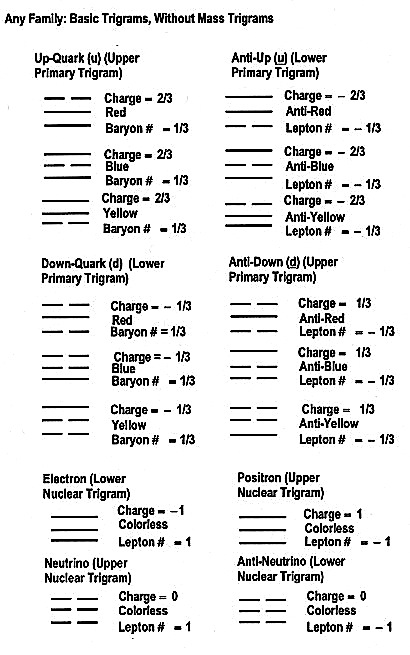DIAGRAM 2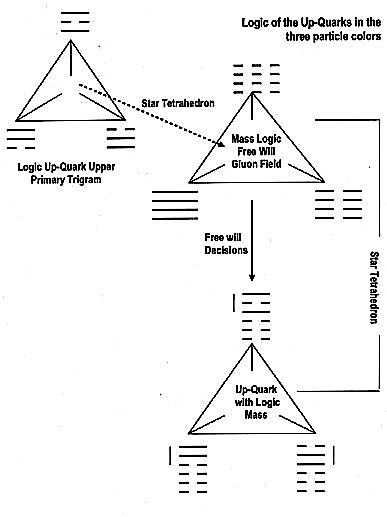DIAGRAM 3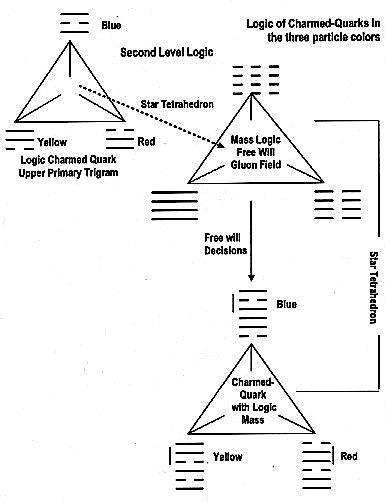DIAGRAM 4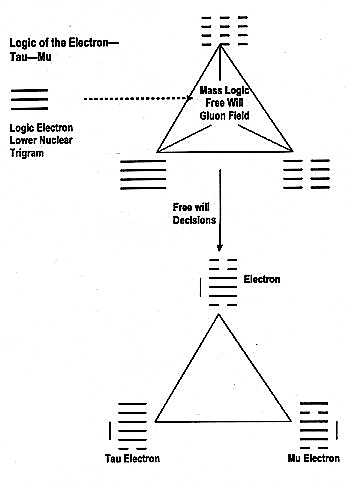DIAGRAM 5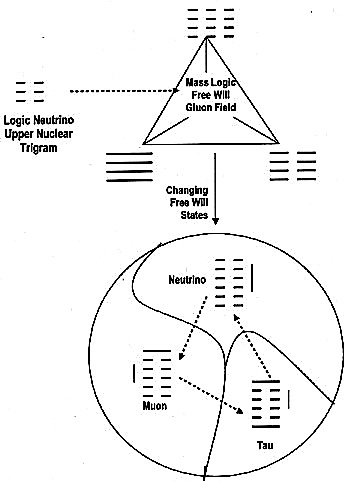Remember, presented in this Website is this author’s self-simulated universe with the attempt to argue that this universe is an infinite set of SELF-Simulated SELF-Encoded Programs within a SELF-Programmed Computer, and that this author’s set is an infinitely small subset. This SELF is GOD, the First Observer, and the Creator of this Computer. Only GOD with HIS infinite wisdom and know how is able to design this Self-Programmable Computer with a set of Involution Logic that automatically guided and integrates all computer programs simulated within. Remember the formula for Matter-Being conservation in Logic-Information; namely: Involution Logic + Evolution Logic + Entropy = 0. Here we have the following mappings: Involution Logic à God’s Logic, Evolution Logic à God’s Logic in Reverse Engineer, Entropy à Mistakes in Reverse Engineer. Be aware, like any computer operations, these Reverse Engineering Mistakes can freeze the computer, or the Universe’s operation, and can even destroy our Universe. This Logic-Information conservation law leads directly to the conservation equation: Logic-Information + Information-Energy + Energy-Mass = 0.